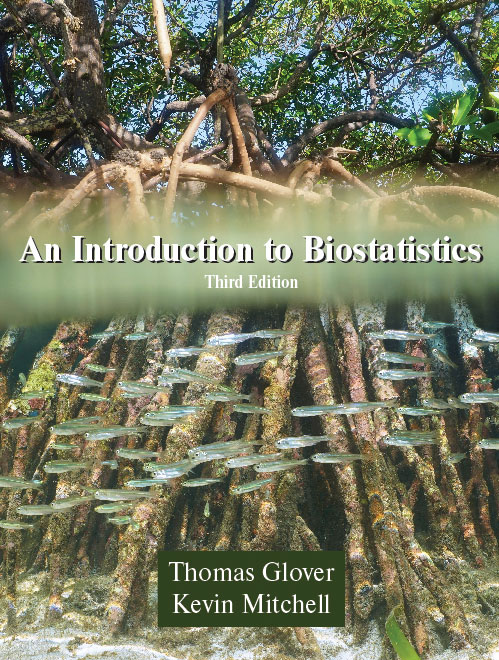539 pages, \$77.95 list
1-4786-2779-4
978-1-4786-2779-1
Student resource materials available here, here, here, and here.
eBook availability
An Introduction to Biostatistics
Third Edition
For over a decade, Glover and Mitchell have provided life-sciences students with an accessible, complete introduction to the use of statistics in their disciplines. The authors emphasize the relationships between probability, probability distributions, and hypothesis testing using both parametric and nonparametric analyses. Copious examples throughout the text apply concepts and theories to real questions faced by researchers in biology, environmental science, biochemistry, and health sciences. Dozens of examples and problems are new to the Third Edition, as are “Concept Checks”—short questions that allow readers to immediately gauge their mastery of the topics presented. Regardless of mathematical background, all readers will appreciate the value of statistics as a fundamental quantitative skill for the life sciences.

300 additional problems are available here.

The authors have prepared a companion guide to using R, a free online statistical tool. Download the guide here.

Data sets (in txt format) to accompany the text's examples and problems can be found here.
Reviews
“The text is well written with abundant, accessible exercises. Very reasonably priced, too.” — Ron Jorgensen, Milwaukee School of Engineering

“Complete, comprehensive, yet simple and basic. Problems are well chosen and the instructors' CD is very helpful.” — Will R. Getz, Fort Valley State University

“We found the book to be accurate and engagingly written. Many of the exercises are accompanied by real datasets. It stood out among the other texts we considered.” — Tom Short, John Carroll University

“The book covers many topics in more depth and with greater clarity than the book I am presently using. I also appreciated the rich, interesting problems at the end of each chapter.” — Dale Zimmerman, University of Iowa

"The book is great. I appreciate the paperback format and like the randomized and extensive problem sets at the end of the chapters.” — Gregg Hartvigsen, SUNY, Geneseo
1. Introduction to Data Analysis
Introduction / Populations and Samples / Variables or Data Types / Measures of Central Tendency: Mean, Median, and Mode / Measures of Dispersion and Variability / Descriptive Statistics for Frequency Tables / The Effect of Coding Data / Tables and Graphs / Quartiles and Box Plots / Accuracy, Precision, and the 30–300 Rule

2. Introduction to Probability
Definitions / Use of Permutations and Combinations / Introduction to Set Theory and Venn Diagrams / Axioms and Rules of Probability / Probability Rules and Mendelian Genetics

3. Probability Distributions
Discrete Random Variables / The Binomial Distribution / The Poisson Distribution / Continuous Random Variables / The Normal Distribution / The Standard Normal Distribution

4. Sampling Distributions
Definitions / Distribution of the Sample Mean / Confidence Intervals for the Population Mean / Confidence Intervals for the Population Variance / Confidence Intervals for a Population Proportion

5. Introduction to Hypothesis Testing
An Overview: The Famous Cornflakes Example / Typical Steps in a Statistical Test of Hypothesis / Type I versus Type II Errors in Hypothesis Testing / Binomial Example of Hypothesis Testing

6. One-Sample Tests of Hypothesis
Hypotheses Involving the Mean (?) / Hypotheses Involving the Variance (?2) / Nonparametric Statistics and Hypothesis Testing / The One-Sample Sign Test / Confidence Intervals Based on the Sign Test / The One-Sample Wilcoxon Signed-Rank Test

7. Tests of Hypothesis Involving Two Samples
Comparing Two Variances / Testing the Difference Between Two Means of Independent Samples / Confidence Intervals for ?1 ? ?2 / The Difference Between Two Means with Paired Data / The Wilcoxon Rank-Sum (Mann-Whitney U) Test / The Sign Test and Paired Data / The Wilcoxon Signed-Rank Test for Paired Data

8. k-Sample Tests of Hypothesis: The Analysis of Variance
Model I ANOVA / Mean Separation Techniques for Model I ANOVAs / Model II ANOVA / The Kruskal-Wallis Test

9. Two-Factor Analysis
Randomized Complete Block Design ANOVA / Factorial Design Two-Way ANOVA / The Friedman k-Sample Test: Matched Data

10. Linear Regression and Correlation
Simple Linear Regression / Simple Linear Correlation Analysis / Correlation Analysis Based on Ranks

11. Goodness of Fit Tests
The Binomial Test / Comparing Two Population Proportions / The Chi-Square Test for Goodness of Fit / The Chi-Square Test for r x k Contingency Tables / The Kolmogorov-Smirnov Test / The Lilliefors Test

Appendix A: Proofs of Selected Results
Appendix B: Answers to Even-Numbered Problems
Appendix C: Tables of Distributions and Critical Values